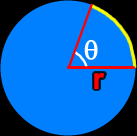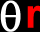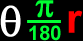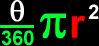# The Trigonometry of Circles

Definitions and formulas for the arc and the arc length of a circle, sector and the area of the sector of a circle, the unit circle, the angles on the unit circle in radians, the angles on the unit circle in degrees, the points on the circumference of the unit circle
Just scroll down or click on what you want and I'll scroll down for you!

 arc of a circle arc length of a circle sector of a circle area of a sector of a circle the unit circle points on the circumferenceof the unit circle

 An arc of a circle:An arc of a circle is a segment of the circumference of the circle.The formula for the arc length of a circle:Arc length of a circle in radians:Arc Length =Arc length of a circle in degrees: Arc Length =A sector of a circle:A sector of a circle is a pie shaped portion of the area of the circle.  Technically, the piece of pie is between two segments coming out of the center of the circle.The area of a sector of a circle:Sector area of a circle in radians:Sector Area =Sector area of a circle in degrees: Sector Area =